Chapter 3. Area Processing via Mask Convolution

3.1 Pixel Neighborhoods

Let (m0,n0) be a pixel position in an image {f(m,n)}. A neighborhood of (m0,n0) is a set of connected (that is, continguous) pixels that contains (m0,n0), which is called the origin of the neighborhood. The most common neighborhoods are rectangles of pxq pixels that contain the pixel (m0,n0) at the center, where p and q are odd numbers so that an exact center exists. Another type of neighborhood is the 3x3 star, which can be considered to be a rectangular neighborhood with the corner points having zero values. Figure 3.1 shows some neighborhoods, but the definition above is rather general and includes a great variety of connected blobs of pixels with one pixel in the aggregate being distinguished as the origin (or "center").

Figure 3.1 Neighborhoods of pixels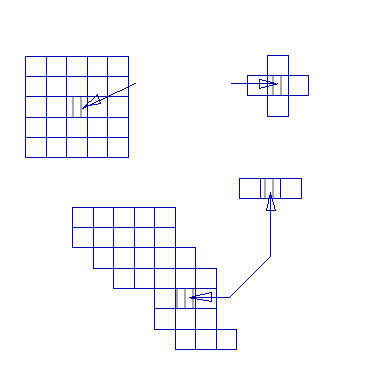Neighborhoods play a central role in image processing. For example, we can smooth an image {f(m,n)} by taking a 3x3 neighborhood of each pixel, in turn, with value fcntr and put the new pixel value gnew to be the average of all the pixel values in that neighborhood. The set of all such new pixels would form a new image {g(m,n)} that would have less detail and wider edges. As another example, we could order the pixel values in each neighborhood of fcntr by magnitude and then pick the middle value as the new value gnew for the origin pixel. This would clear the image of small dots of strongly differing shades of gray (outliers) from the typical ones in the neighborhood. In general, for each original pixel we consider a neighborhood of it and map the pixel values in that neighborhood into a new grayscale value.

Convolution. Figure 3.2 presents the convolution operation for a pxq = 3x3 mask {h(i,j): 0 i,j 2} of weights for 3x3 neighborhoods of the same size. We also denote the mask by {h0,...hpq-1} for convenience. For a rectangular pxq mask, both p and q are odd integers so that there is a center pixel with value fc (and pixel values f0,...,fc,...,fr) in any pxq rectangular neighborhood, where r = pq-1. On each neighborhood the operation computes a new pixel value gc in place of fc for the output image file using

gc = h0f0 + ... + hrfr  	(3.1)
<

Note that for a 3x3 neighborhood and mask, f4 = fc, g4 = gc and h4 = hc. The convolution operation is defined over the entire image by its definition on each pixel neighborhood and is denoted by "*" in the literature. Convolution, which transforms an image {f(m,n)} into a new image {g(m,n)} is denoted by

{g(m,n)} = {h(i,j)}*{f(m,n)}		                                        (3.2)
by convolving the fixed mask {h(i,j)} with the neighborhood of every pixel in {f(m,n)}.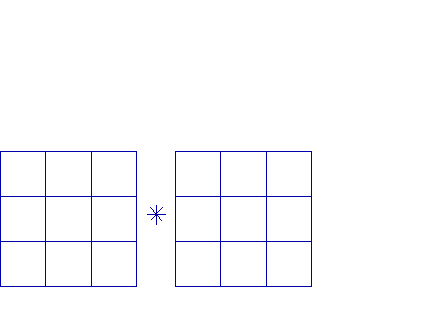Figure 3.2. Convolution with a 3x3 Mask
The mask and neighborhood size are fixed at some pxq for any particular convolution, where p and q are odd positive integers. The process starts at the upper lefthand corner of the image and processes one pixel at a time via the mask convolution on the pixel's neighborhood. After the top left (first) pixel is processed to obtain a new output pixel value gc, the process then steps to the right by one pixel and convolves the mask with that pixel's neighborhood to obtain an output pixel. When the last pixel in a row of pixels has been processed, the mask comes back to the left side of the image and moves down one pixel and processes the next row of image pixels similarly. This is repeated until all rows of pixels in the image {f(m,n)} have been processed. Section 3.6 provides an algorithm for convolution.

The pixels at the outer edges of the image need not be processed (the neighborhoods of these pixel positions do not fit inside the image), or else only the mask entries that intersect the image are used. Another technique is to repeat the boundary pixels outside the boundaries sufficiently many times to complete the pxq neighborhood of the mask size.

The Identity Convolution Mask. The most basic convolution mask is the pxq identity operator, which yields the pixel value gc = fc on each neighborhood. The pxq identity mask contains all zero except the center, which is unity. For example, the 3x3 identity mask operates on a 3x3 neighborhood via

0  0  0		f0  f1  f2

gc = 0 1 0 * f3 f4 f5 = {(j4) (0)fj} + (1)f4 = f4 = fc (3.3)

0 0 0 f6 f7 f8

Averaging Convolution Masks. Averaging is the next most basic operation and is sometimes useful for smoothing noise or for blurring. Each new pixel gc in the output image is the weighted average of the pixels in the corresponding pxq neighborhood of fc. The simplest averaging convolution mask weights each neighborhood pixel equally and uses a mask factor per

1 1 1 f0 f1 f2

(1/9) 1 1 1 * f3 f4 f5 = (1/9)Sum(j=0,8) fj (3.4)

1 1 1 f6 f7 f8

The central pixel is often weighted more than the others, and the others may be weighted unequally for different degrees of smoothing. Examples of other averaging convolution masks and their mask factors are

1 1 1 f0 f1 f2

(1/10) 1 2 1 * f3 f4 f5 = (1/10){[Sum(j4) fj ] + 2f4} (3.5a)

1 1 1 f6 f7 f8

1 2 1 f0 f1 f2

(1/16) 2 4 2 * f3 f4 f5 = {f0+f2+f6+f8 + 2[f1+f3+f5+f7] + 4f4}/16 (3.5b)

1 2 1 f6 f7 f8

Figure 3.3. Original "Lena" Figure 3.4. Blurred "Lena"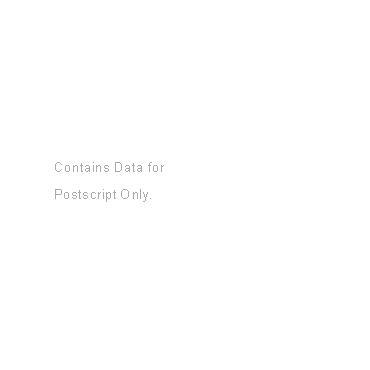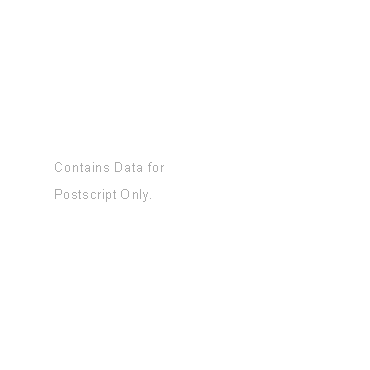Averaging masks that smooth lightly and very lightly are

0 1 0 0 1 0

(1/5) 1 1 1 (1/6) 1 2 1

0 1 0 0 1 0

Blurring Masks. It is a sometimes useful effect to blur an image by oversmoothing it by averaging the neighborhood pixel values not adjacent to the center pixel. Figure 3.3 shows the original "Lena" while Figure 3.4 shows the blurred result obtained with the lefthand 5x5 mask of the two examples given below.

1 1 1 1 1 0 1 1 1 1 1 0

1 0 0 0 1 1 1 1 1 1 1 1

(1/16) 1 0 0 0 1 1 1 0 0 0 1 1

1 0 0 0 1 (1/36) 1 1 0 0 0 1 1

1 1 1 1 1 1 1 0 0 0 1 1

1 1 1 1 1 1 1

0 1 1 1 1 1 0

3.3 Smoothing with Medians and Trimed Medians

We have already seen convolution masks that average, which is one form of smoothing. This is useful for eliminating low level noise or giving skin a smooth look in a photographic portrait. However, averaging is strongly affected by outlier pixel values, which is the "snake swallowing the egg" (SSTE) syndrome. An outlier causes the average to be in error, and as the mask moves to new centers nearby, the outlier remains in the new neighborhoods so that the outlier strongly influences (erroneously) a block section of the image larger than the mask. Actually, any pxq mask maps, or transforms, a single outlier into a (2p-1)x(2q-1) block of erroneous values.

A well known method for avoiding the SSTE syndrome is to use the median (order statistics). For each pixel and neighborhood centered on it, we order the neighborhood pixel values from smallest to largest. The indexing by order is designated by

fj(0) fj(1) ... fj(pq-1) (3.6)

There are two ways to proceed here. The first is to take the middle pixel value, fj(c), where c is the number of the center pixel, and put

gc = fj(c) (3.7)

This is the middle of all the actual gray level values of pixels in the neighborhood. This is often a good way to remove speckle (also called salt and pepper) noise, which appears as pixel size dots of black or white on the image.

The median of a neighborhood is not always the value that best portrays the image information. For this reason, good results are sometimes obtained by using the -trimmed median. To obtain this value, we set to be 1 or 2 or so, throw out the lowest and highest pixel values and average the remaining pq - 2 pixel values to obtain gc.

Median processing does not blur the image. It preserves edges rather than smear them as does averaging. The -trimmed median also has less blurring and spreading of edges. Thus, these are powerful processes for smoothing noise from the image while preserving its features.

A process known as unsharp masking (taken from the photographic processing industry) first smooths (blurs) an image and then the subtracts the smoothed image from the original image. The smoothing yields an image whose variation (rates of change) in gray levels across the spatial dimensions is lowered. Smoothing actually spreads out the changes at all rates into more gradual changes in grayscale across the dimensions m and n. When the blurred image is subtracted, it leaves the more rapid variations (changes at a higher rate). The resultant image appears sharp, that is, the edges are narrower and have greater contrast on each side. The average gray level is reduced by subtraction, so we need to multiply the original image by a factor ß > 1.0 to increase its intensity, or brightness power.

The two operations of blurring and subtracting from the original can be combined into a single convolution. We first increase the brightness power by multiplying the identity convolution mask by the gain ß > 1.0, which is called boosting the original.

0 0 0 0 0 0 0 0 0 0

0 0 0 0 0 0 0 0 0 0

(ß) 0 0 1 0 0 = 0 0 ß 0 0 (3.8)

0 0 0 0 0 0 0 0 0 0

0 0 0 0 0 0 0 0 0 0

Then we subtract an averaging mask that blurs the image. Using a 5x5 mask example, the resulting unsharp masking convolution mask is obtained via

0 0 0 0 0 1 1 1 1 1 -1 -1 -1 -1 -1

0 0 0 0 0 1 1 1 1 1 -1 -1 -1 -1 -1

0 0 ß 0 0 - (1/26) 1 1 2 1 1 = (1/26) -1 -1 (26ß-2) -1 -1 (3.9)

0 0 0 0 0 1 1 1 1 1 -1 -1 -1 -1 -1

0 0 0 0 0 1 1 1 1 1 -1 -1 -1 -1 -1

A gain ß of approximately 2.0 is often satisfactory, but the best value depends upon the average brightness power (1.2 may be quite good). Figure 3.5 shows the original "Shuttle" image, while Figure 3.6 shows the smoothed version that was subtracted from the boosted original (ß = 2) and Figure 3.7 presents the resulting unsharp-masked image.

The sum of all mask entries multiplied by the mask factor in Equation (3.8) (in this case it is 1/26) should equal unity to keep the output average brightness the same as in the original image. If the sum of the weights is greater than 1 (respectively, less than 1) then the resultant image will be brighter (respectively, darker) than the original image.

Figure 3.5. Orginal "shuttle" Figure 3.6. Smoothed "shuttle"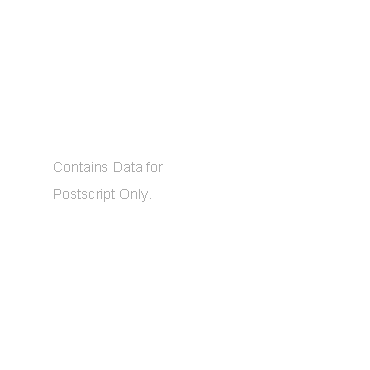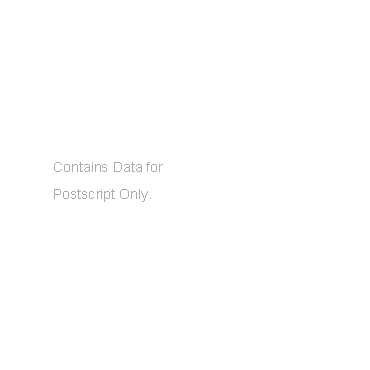Figure 3.7. Unsharp-masked "shuttle"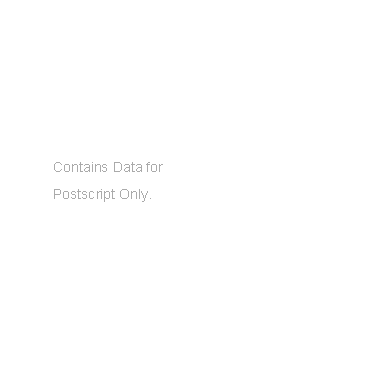3.5 Sharpening and Detecting Edges

Directional Derivative Edge Detectors. We have seen that unsharp masking by subtracting a smoothed image is an effective way to sharpen an image. The underlying process of sharpening must make edges more exaggerated, that is, thinner with a greater difference between the darker and lighter pixels along the edges. To detect the edges, it is necessary to obtain the differences in each neighborhood along the horizontal (y), vertical (x) and two diagonal directions (y = -x and y = x). Let a neighborhood of (m,n) have the values

f(m-1,n-1) f(m-1,n) f(m-1,n+1)

f(m,n-1) f(m,n) f(m,n+1)

f(m+1,n-1) f(m+1,n) f(m+1,n+1)

Figure 3.8 displays a sharp edge, while Figure 3.9 presents a smooth edge. The first can be considered a sharpening of the second and likewise the second can be considered to be a smoothing of the first. Edges in real world images are usually wider than the one shown in Figure 3.8.